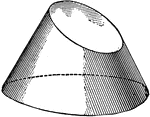### Intersection Of A Plane And Cone

Illustration showing the intersection of a plane with a cone.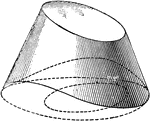### Intersection Of A Plane And Cone

Illustration showing the intersection of a plane with a cone. The solid is shown in perspective and…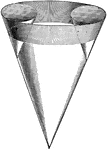### 3 Intersecting Cones

Illustration of the intersection of 3 cones.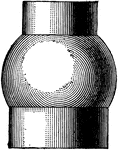### Intersecting Cylinder and Sphere

Illustration of the intersection of a cylinder and a sphere.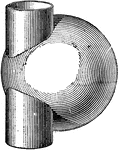### Intersecting Cylinder and Sphere

Illustration of the intersection of a cylinder and a sphere.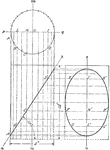### Plane Intersecting A Cylinder

Illustration of a cylinder cut by a plane making an angle of 57° with the base.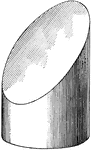### Plane Intersecting Cylinder

Illustration showing the intersection of a plane with a cylinder.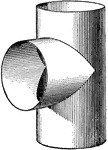### 2 Intersecting Cylinders

Illustration showing the intersection of two intersecting cylinders.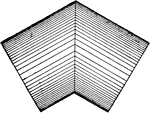### Intersecting Cylinders

Illustration of the intersection of 2 cylinders of equal diameter.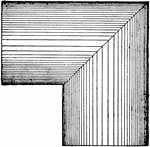### Intersecting Cylinders

Illustration of the intersection of 2 cylinders of equal diameter.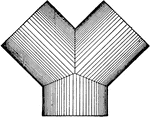### Intersecting Cylinders

Illustration of the intersection of 3 cylinders of equal diameter.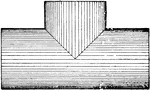### Intersecting Cylinders

Illustration of the intersection of 2 cylinders of equal diameter.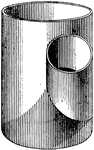### Intersecting Cylinders

Illustration of the intersection of 2 cylinders of unequal diameter.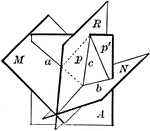### Plane Bisecting Dihedral Angle

Diagram used to prove the theorem: "Every point in a plane which bisects a dihedral angle is equidistant…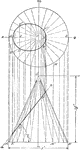### Conic Section Ellipse

Illustration of a cone cut by a plane that is not parallel to the base and does not intersect the base.### Conic Section Hyperbola

Illustration of a cone cut by a plane parallel to the axis of the cone and perpendicular to the vertical…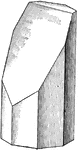### Plane Intersecting Octagonal Prism

Illustration showing the intersection of a plane with an octagonal prism.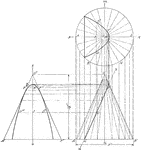### Conic Section Parabola

Illustration of a cone cut by a plane that is parallel to one of the elements of the cone. The curve…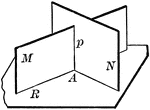### 2 Intersecting Planes Perpendicular To A Third Plane

"If two intersecting planes are each perpendicular to a third plane, their intersection is also perpendicular…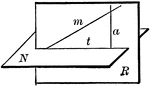### Plane Passed Perpendicular To A Given Plane

"Through a given line oblique to a plane, one, and only one plane, can be passed perpendicular to the…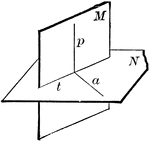### Perpendicular Planes

"If two planes are perpendicular to each other, a line in one of them perpendicular to the intersection…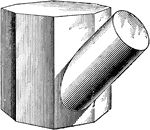### Intersecting Prism and Cylinder

Illustration of the intersection of an octagonal prism and a cylinder. The figures intersect at an oblique…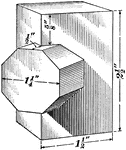### Intersecting Prisms

Illustration of the intersection of a quadrangular prism and an octagonal prism. The figures intersect…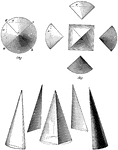### Relation Of Pyramid To Cone

Illustration showing the cone being treated as a many-sided pyramid.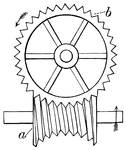### Hindley's Screw

"A screw cut on a solid, of such form that if any plane be taken through its longitudinal axis, the…